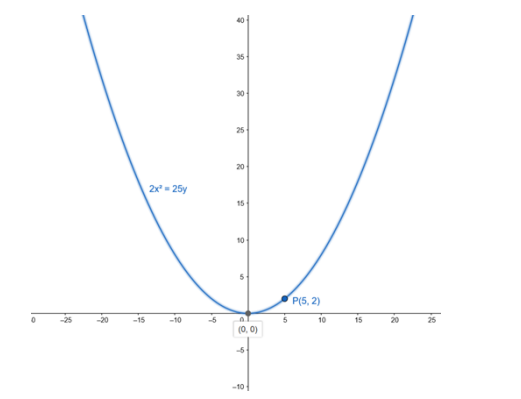# Find the equation of the parabola with vertex at the origin, passing through

Question:

Find the equation of the parabola with vertex at the origin, passing through the point P(5, 2) and symmetric with respect to the y-axis.

Solution:

The equation of a parabola with vertex at the origin and symmetric about the y-axis is

$x^{2}=4 a y$

Since point P(5,2) passes through above parabola we can write,

$5^{2}=4 a(2)$

$\cdot 25=8 a$

$a=\frac{25}{8}$

Therefore, the equation of a parabola is

$x^{2}=4 \cdot \frac{25}{8} y$

$x^{2}=\frac{25}{2} y$

- $2 x^{2}=25 y$